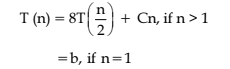# UGC-NET | UGC-NET CS 2017 Nov – III | Question 33

Consider the recurrence relation:Where b and c are constants.
The order of the algorithm corrosponding to above recurrence relation is:
(A) n
(B) n2
(C) n log n
(D) n3

Explanation: We can use Master theorem to solve this recurrance relation:

```T(n) = aT(n/2) + Θ(nklogpn)
In given question:
T(n) = 8T(n/2) + Cn
here a = 8 and b = 2 and k = 1.
clearly a > bk
So T(n) = Θ(nlogba )
T(n) = Θ(nlog2 8)
ie T(n) = Θ(n3)
```

So, option (D) is correct.

Quiz of this Question

My Personal Notes arrow_drop_up
Article Tags :

Be the First to upvote.

Please write to us at contribute@geeksforgeeks.org to report any issue with the above content.